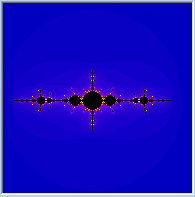## Thursday, June 2, 2011

### The Mandelbrot SetThe Mandelbrot Set
Ok, on we go to the fractal design that probably led you here in the first place... The famous Mandelbrot set.

So you know now that there are as many Julia sets as there are complex numbers (an infinite amount), and each set is generated according to the results of iterating the function f(x) = x² + c ... So in other words, for each and every Julia set, there is a corresponding formula, each having a unique value of c (either a real, imaginary, or complex number).

Did you play around with Fractal eXtreme as I suggested in the previous post? Really cool, right? But I bet you felt a bit restricted in terms of which numbers generated the coolest Julia sets. You may have noticed that entering anything under -2 in the X field didn't do anything too interesting. And the same for above 1. And in the Y field, you're were even more restricted. Anything above 1.4 or so, and under -1.4, hardly produced anything worth calling a fractal. This is because, as I explained in the previous post, Julia sets are coloured according to how fast all the points on their complex plane map out or "escape" to infinity. So when your c value (real,imaginary or complex number) is too big, obviously every point mapped with our function f(x) = x² + c escapes to infinity very very quickly, and thus, the image is coloured mostly monochromatic, (blue in the case of Fractal eXtreme's default colour scheme).

Ok, to continue... Take a look at these Julia set examples:f(z)= z² + (0.3,0.01i)f(z)= z² + (0.18,0.7i)f(z)= z² + (-1.36,-0.05i)f(z)= z² + (0.3,0.25i)f(z)= z² + (-1.3,0i)f(z)= z² + (-0.71,-0.17i)

Notice how some of them have large black areas in them, and some of them don't have any black in them at all. From the previous post, you know that the black area represents every pointon the complex plane whose fate it is to stay bounded when mapped with our function, and all the rest of the points, in whatever colour, escape to infinity.

What you need to know is, every Julia set can be classified as either connected (have a single black area, of which the point zero is included) or disconnected (no black area at all; or sporadic black areas, with the point 0 not included).

To determine mathematically whether a Julia set is connected or disconnected, you have to take its corresponding function (with unique c value), make z = 0 and iterate it forever. If the point stays bounded, the Julia is connected, but if the point escapes to infinity, the Julia is disconnected.

Using the first two Julias above, let me demonstrate this as well as I can:

f(z)= z² + (0.3,0.25i) .... make z=0
f(0)= 0 + (0.3,0.25i)
f(0.3,0.25i)= (0.3,0.25i)² + (0.3,0.25i) = (0.3275 + 0.4i)
f(0.3275 + 0.65) = (0.3275 + 0.65)² + (0.3,0.25i) = (-0.01524375 + 0.67575i)
etc

And the second Julia set example:

f(z)= z² + (0.3,0.01i) .... again, make z = 0
f(0)= 0² + (0.3,0.01i)
f(0.3,0.01i)= (0.3,0.01i)² + (0.3,0.01i)= (0.3899 + 0.016)
f(0.3899 + 0.016)= (0.3899 + 0.016)² + (0.3,0.01i) = (0.6899 + 0.026)
etc

Forgive me for being lazy and not working out further iterations but the following images, with the path of the mapped points shown with a white line, show clearly how the first becomes bound in a spiral, but the second escapes very quickly to infinity. You can use Fractal eXtreme to test out the theorum on any Julia you like.Here you can see the location of each example Julia I used above:

And that's how the Mandelbrot set is generated.

Hehehe... How? you ask. It's like this:

Each and every Julia set has a corresponding point on the complex plane, right? And all Julias are classified as either connected or disconnected, as determined by the method above. Well, in the same way the Julia's are generated with every single point being coloured depending on their "fates", so the Mandelbrot Set is generated depending on whether each point's corresponding Julia is connected or disconnected. If it is connected, the point is coloured black, and if disconnected, is coloured some other colour, based on "how fast" the 0 point of the Julia escapes to infinity.

Understand? I hope so! :P ... Teaching all this is much harder than it was to learn, I must admit. Go through the post a few times if you haven't quite got it yet. And if all else fails, use fractalmath's original video to help you. I learnt everything from him :) :

1."the Mandelbrot Set is generated depending on whether each point's corresponding Julia is connected or disconnected. If it is disconnected, the point is coloured black, and if connected, is coloured some other colour, based on "how fast" the 0 point of the Julia escapes to infinity".

Sorry, but as I understand it's exactly the opposite that's the basis of the coloring of the points in question!
(Ie: the connected are colored black!)

Kind regards from Jens in Brussels.

2.Oh damn.. Quite right! The rest of my explanation is sound, just got mixed up in that sentence... Will correct it immediately.

Thank you :) I hope you've been reading and enjoying the rest of the blog :) It gets truly wonderous after this page.

3.check the video by "The Mathemagicians' Guild"

https://youtu.be/7MotVcGvFMg

4.https://youtu.be/ovJcsL7vyrk

must watch